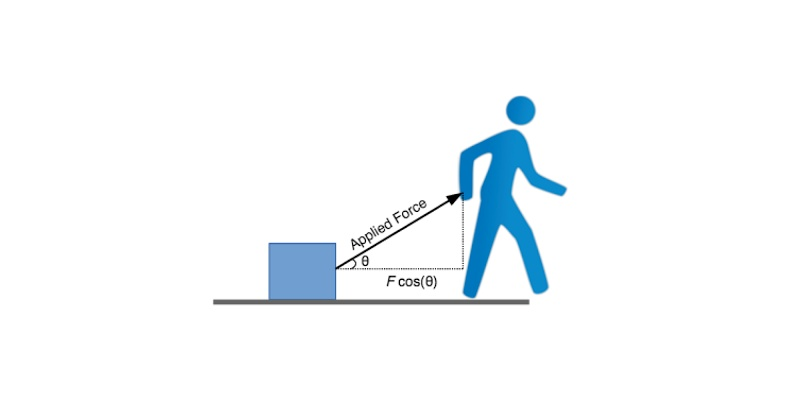### Work And Energy Trivia Quiz For 9th Grade Students### Questions & Options

#### 1. The SI unit of work is ....

 132795 Joule 132796 Kilogram 132797 Meter 132798 None of these

#### 2. Mechanical energy is the ....

 132799 Product of Kinetic energy and Potential energy 132800 Sum of Kinetic energy and Potential energy 132801 Both of these 132802 None of these

#### 3. Objects in motion possess energy this kind of energy is ....

 132803 Potential energy 132804 Kinetic energy 132805 Both of these 132806 None of these

#### 4. When two identical bodies are in motion then the body with a higher velocity has more ......

 132807 Kinetic energy 132808 Potential energy 132809 Mechanical energy 132810 None of these

#### 5. Which one of the following is not a factor which affects kinetic energy?

 132811 Mass 132812 Momentum 132813 Velocity 132814 Time

#### 6. How many types of potential energy?

 132815 Two 132816 Three 132817 Four 132818 Five

#### 7. Who gave the law of conservation of energy?

 132819 Antoine Lavoisier's 132820 Sir Isaac Newton 132821 Julius Mayer 132822 None of these

#### 8. What is the SI unit of power?

 132823 Joule 132824 Watt 132825 Meter 132826 Kilogram

#### 9. When both the force and the displacement are in the same direction, work done is ....

 132827 Negative 132828 Positive 132829 Nuetral 132830 None of these

#### 10. If force and displacement act at an angle of 90° then work done is.....

 132831 Positive 132832 Negative 132833 Zero 132834 None of these

#### 11. Which type of energy is possessed by a body due to its change in shape?

 132835 Gravitational Potential Energy 132836 Elastic Potential Energy 132837 Chemical Potential Energy 132838 None of these